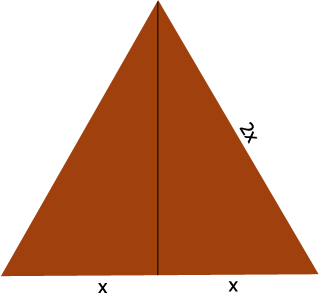SEARCH HOMEMath Central Quandaries & QueriesQuestion from shreyaarora, a student: if the area of on equilateral triangle is 24/3cm,then what is its perimeterHi Shreyaarora,

Suppose each side of the triangle is $2x$ centimeters long.What is the height of the triangle? (Ask Pythagoras.) What is an expression for the area of the triangle. But you know the area of the triangle. Solve for $x.$

PennyMath Central is supported by the University of Regina and The Pacific Institute for the Mathematical Sciences.